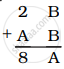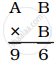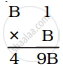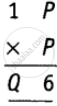# NCERT solutions for Mathematics Exemplar Class 8 chapter 13 - Playing With Numbers [Latest edition]

#### Chapters## Chapter 13: Playing With Numbers

Exercise
Exercise [Pages 408 - 413]

### NCERT solutions for Mathematics Exemplar Class 8 Chapter 13 Playing With Numbers Exercise [Pages 408 - 413]

#### Choose the correct alternative:

Exercise | Q 1 | Page 408

Generalised form of a four-digit number abdc is ______.

• 1000a + 100b + 10c + d

• 1000a + 10c + 10b + d

• 1000a + 100b + 10d + c

• a × b × c × d

Exercise | Q 2 | Page 409

Generalised form of a two-digit number xy is ______.

• x + y

• 10x + y

• 10x – y

• 10y + x

Exercise | Q 3 | Page 409

The usual form of 1000a + 10b + c is ______.

• abc

• abc0

• a0bc

• ab0c

Exercise | Q 4 | Page 409

Let abc be a three-digit number. Then abc – cba is not divisible by ______.

• 9

• 11

• 18

• 33

Exercise | Q 5 | Page 409

The sum of all the numbers formed by the digits x, y and z of the number xyz is divisible by ______.

• 11

• 33

• 37

• 74

Exercise | Q 6 | Page 409

A four-digit number aabb is divisible by 55. Then possible value(s) of b is/are ______.

• 0 and 2

• 2 and 5

• 0 and 5

• 7

Exercise | Q 7 | Page 409

Let abc be a three digit number. Then abc + bca + cab is not divisible by ______.

• a + b + c

• 3

• 37

• 9

Exercise | Q 8 | Page 409

A four-digit number 4ab5 is divisible by 55. Then the value of b – a is ______.

• 0

• 1

• 4

• 5

Exercise | Q 9 | Page 409

If abc is a three-digit number, then the number abc – a – b – c is divisible by ______.

• 9

• 90

• 10

• 11

Exercise | Q 10 | Page 409

A six-digit number is formed by repeating a three-digit number. For example 256256, 678678, etc. Any number of this form is divisible by ______.

• 7 only

• 11 only

• 13 only

• 1001

Exercise | Q 11 | Page 409

If the sum of digits of a number is divisible by three, then the number is always divisible by ______.

• 2

• 3

• 6

• 9

Exercise | Q 12 | Page 409

If x + y + z = 6 and z is an odd digit, then the three-digit number xyz is ______.

• an odd multiple of 3

• odd multiple of 6

• even multiple of 3

• even multiple of 9

Exercise | Q 13 | Page 410

If 5A + B3 = 65, then the value of A and B is ______.

• A = 2, B = 3

• A = 3, B = 2

• A = 2, B = 1

• A = 1, B = 2

Exercise | Q 14 | Page 410

If A3 + 8B = 150, then the value of A + B is ______.

• 13

• 12

• 17

• 15

Exercise | Q 15 | Page 410

If 5A × A = 399, then the value of A is ______.

• 3

• 6

• 7

• 9

Exercise | Q 16 | Page 410

If 6A × B = A 8 B, then the value of A – B is ______.

• –2

• 2

• –3

• 3

Exercise | Q 17 | Page 410

Which of the following numbers is divisible by 99?

• 913462

• 114345

• 135792

• 3572406

#### Fill in the blank:

Exercise | Q 18 | Page 410

3134673 is divisible by 3 and ______.

Exercise | Q 19 | Page 410

20 × 3 is a multiple of 3 if the digit × is ______ or ______ or ______.

Exercise | Q 20 | Page 410

3 × 5 is divisible by 9 if the digit x is ______.

Exercise | Q 21 | Page 410

The sum of a two-digit number and the number obtained by reversing the digits is always divisible by ______.

Exercise | Q 22 | Page 410

The difference of a two-digit number and the number obtained by reversing its digits is always divisible by ______.

Exercise | Q 23 | Page 410

The difference of three-digit number and the number obtained by putting the digits in reverse order is always divisible by 9 and ______.

Exercise | Q 24 | Page 410

Ifthen A = ______ and B = ______.

Exercise | Q 25 | Page 410

Ifthen A = ______ and B = ______.

Exercise | Q 26 | Page 410

Ifthen B = ______.

Exercise | Q 27 | Page 411

1 × 35 is divisible by 9 if x = ______.

Exercise | Q 28 | Page 411

A four-digit number abcd is divisible by 11, if d + b = ______ or _____.

Exercise | Q 29 | Page 411

A number is divisible by 11 if the differences between the sum of digits at its odd places and that of digits at the even places is either 0 or divisible by ______.

Exercise | Q 30 | Page 411

If a 3-digit number abc is divisible by 11, then ______ is either 0 or multiple of 11.

Exercise | Q 31 | Page 411

If A × 3 = 1A, then A = ______.

Exercise | Q 32 | Page 411

If B × B = AB, then either A = 2, B = 5 or A = ______, B = ______.

Exercise | Q 33 | Page 411

If the digit 1 is placed after a 2-digit number whose tens is t and ones digit is u, the new number is ______.

#### State whether the following statement is True or False:

Exercise | Q 34 | Page 411

A two-digit number ab is always divisible by 2 if b is an even number.

• True

• False

Exercise | Q 35 | Page 411

A three-digit number abc is divisible by 5 if c is an even number.

• True

• False

Exercise | Q 36 | Page 411

A four-digit number abcd is divisible by 4 if ab is divisible by 4.

• True

• False

Exercise | Q 37 | Page 411

A three-digit number abc is divisible by 6 if c is an even number and a + b + c is a multiple of 3.

• True

• False

Exercise | Q 38 | Page 411

Number of the form 3N + 2 will leave remainder 2 when divided by 3.

• True

• False

Exercise | Q 39 | Page 411

Number 7N + 1 will leave remainder 1 when divided by 7.

• True

• False

Exercise | Q 40 | Page 411

If a number a is divisible by b, then it must be divisible by each factor of b.

• True

• False

Exercise | Q 41 | Page 411

If AB × 4 = 192, then A + B = 7.

• True

• False

Exercise | Q 42 | Page 411

If AB + 7C = 102, where B ≠ 0, C ≠ 0, then A + B + C = 14

• True

• False

Exercise | Q 43 | Page 411

If 213 × 27 is divisible by 9, then the value of x is 0.

• True

• False

Exercise | Q 44 | Page 411

If N ÷ 5 leaves remainder 3 and N ÷ 2 leaves remainder 0, then N ÷ 10 leaves remainder 4.

• True

• False

#### Solve the following:

Exercise | Q 45 | Page 411

Find the least value that must be given to number a so that the number 91876a2 is divisible by 8.

Exercise | Q 46 | Page 412

Ifwhere Q – P = 3, then find the values of P and Q.

Exercise | Q 47 | Page 412

If 1AB + CCA = 697 and there is no carry–over in addition, find the value of A + B + C.

Exercise | Q 48 | Page 412

A five-digit number AABAA is divisible by 33. Write all the numbers of this form.

Exercise | Q 49 | Page 412

Find the value of the letter in the following questions.

A   A
+A   A
XA  Z

Exercise | Q 50 | Page 412

Find the value of the letter in the following questions.

8  5
+ 4  A
B C 3

Exercise | Q 51 | Page 412

Find the value of the letter in the following questions.

B  6
+ 8  A
C A 2

Exercise | Q 52 | Page 412

Find the value of the letter in the following questions.

1  B  A
+ A  B  A
8   B  2

Exercise | Q 53 | Page 412

Find the value of the letter in the following questions.

C  B  A
+ C  B  A
1A  3  0

Exercise | Q 54 | Page 412

Find the value of the letter in the following questions.

B  A  A
+ B  A  A
3   A  8

Exercise | Q 55 | Page 412

Find the value of the letter in the following questions.

A  0  1  B
+ 1  0  A  B
B  1  0  8

Exercise | Q 56 | Page 412

Find the value of the letter in the following questions.

A  B
×      6
C 6 8

Exercise | Q 57 | Page 412

Find the value of the letter in the following questions.

A  B
× A  B
6 A  B

Exercise | Q 58 | Page 412

Find the value of the letter in the following questions.

A  A
×  A
C A  B

Exercise | Q 59 | Page 412

Find the value of the letter in the following questions.

A  B
– B  7
4  5

Exercise | Q 60 | Page 412

Find the value of the letter in the following questions.

8  A  B  C
– A  B  C  5
D  4   8  8

Exercise | Q 61 | Page 412

If 2A7 ÷ A = 33, then find the and B – A = 1

Exercise | Q 62 | Page 412

212 × 5 is a multiple of 3 and 11. Find the value of x.

Exercise | Q 63 | Page 412

Find the value of k where 31k 2 is divisible by 6.

Exercise | Q 64 | Page 412

1y3y6 is divisible by 11. Find the value of y.

Exercise | Q 65 | Page 412

756 x is a multiple of 11, find the value of x.

Exercise | Q 66 | Page 412

A three-digit number 2 a 3 is added to the number 326 to give a three-digit number 5b9 which is divisible by 9. Find the value of b – a.

Exercise | Q 67 | Page 413

Let E = 3, B = 7 and A = 4. Find the other digits in the sum

B A S E
+ B A L L
G A M E S

Exercise | Q 68 | Page 413

Let D = 3, L = 7 and A = 8. Find the other digits in the sum

M A D
+ A  S
+     A
B U L L

Exercise | Q 69 | Page 413

If from a two-digit number, we subtract the number formed by reversing its digits then the result so obtained is a perfect cube. How many such numbers are possible? Write all of them.

Exercise | Q 70.(a) | Page 413

Work out the following multiplication.

12345679
×   9

Use the result to answer the following questions.

What will be 12345679 × 45?

Exercise | Q 70.(b) | Page 413

Work out the following multiplication.

12345679
×   9

Use the result to answer the following questions.

What will be 12345679 × 63?

Exercise | Q 70.(c) | Page 413

Work out the following multiplication.

12345679
×    9

Use the result to answer the following questions.
By what number should 12345679 be multiplied to get 888888888?

Exercise | Q 70.(d) | Page 413

Work out the following multiplication.

12345679
×    9

Use the result to answer the following questions.
By what number should 12345679 be multiplied to get 999999999?

Exercise | Q 71.(i) | Page 413

Find the value of the letters in the following:

PQ
× 6
QQQ

Exercise | Q 71.(ii) | Page 413

Find the value of the letters in the following:

2  L M
+  L M  1
M  1  8

Exercise | Q 72 | Page 413

If 148101B095 is divisible by 33, find the value of B.

Exercise | Q 73 | Page 413

If 123123A4 is divisible by 11, find the value of A.

Exercise | Q 74 | Page 413

If 56 × 32y is divisible by 18, find the least value of y.

## Chapter 13: Playing With Numbers

Exercise## NCERT solutions for Mathematics Exemplar Class 8 chapter 13 - Playing With Numbers

NCERT solutions for Mathematics Exemplar Class 8 chapter 13 (Playing With Numbers) include all questions with solution and detail explanation. This will clear students doubts about any question and improve application skills while preparing for board exams. The detailed, step-by-step solutions will help you understand the concepts better and clear your confusions, if any. Shaalaa.com has the CBSE Mathematics Exemplar Class 8 solutions in a manner that help students grasp basic concepts better and faster.

Further, we at Shaalaa.com provide such solutions so that students can prepare for written exams. NCERT textbook solutions can be a core help for self-study and acts as a perfect self-help guidance for students.

Concepts covered in Mathematics Exemplar Class 8 chapter 13 Playing With Numbers are Numbers in General Form, Games with Numbers, Letters for Digits, Divisibility by 2, Divisibility by 3, Divisibility by 10, Divisibility by 9, Divisibility by 5.

Using NCERT Class 8 solutions Playing With Numbers exercise by students are an easy way to prepare for the exams, as they involve solutions arranged chapter-wise also page wise. The questions involved in NCERT Solutions are important questions that can be asked in the final exam. Maximum students of CBSE Class 8 prefer NCERT Textbook Solutions to score more in exam.

Get the free view of chapter 13 Playing With Numbers Class 8 extra questions for Mathematics Exemplar Class 8 and can use Shaalaa.com to keep it handy for your exam preparation